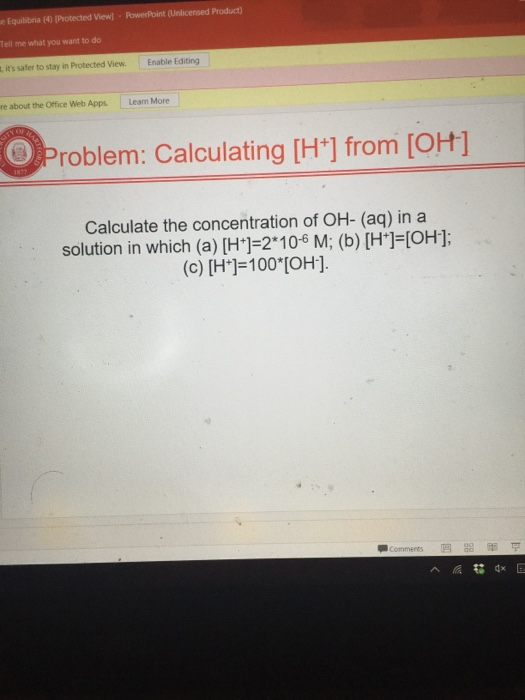# Question & Answer: Calculate the concentration of OH- (aq) in a solution in which (a) [H^+] = 2*10^-6 M…..Calculate the concentration of OH- (aq) in a solution in which (a) [H^+] = 2*10^-6 M: (b) [H^+] = [OH^-]: (c) [H^+] = 100*[OH^-].